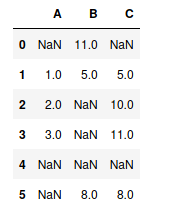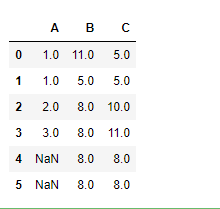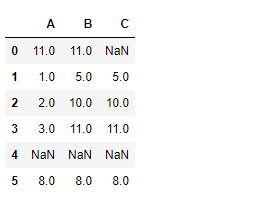# Python | Pandas dataframe.bfill()

• Last Updated : 13 Feb, 2020

Python is a great language for doing data analysis, primarily because of the fantastic ecosystem of data-centric python packages. Pandas is one of those packages and makes importing and analyzing data much easier.

Pandas` dataframe.bfill()` is used to backward fill the missing values in the dataset. It will backward fill the `NaN` values that are present in the pandas dataframe.

Syntax: DataFrame.bfill(axis=None, inplace=False, limit=None, downcast=None)

Parameters:
axis : ‘rows’ or ‘columns’
inplace : boolean, default False
limit : integer value, No. of consecutive `na` cells to be populated.

Example #1: Use `bfill()` function to populate missing values `na` values in the dataframe across rows.

 `# importing pandas as pd``import` `pandas as pd`` ` `# Creating a dataframe with "na" values.`` ` `df ``=` `pd.DataFrame({``"A"``:[``None``, ``1``, ``2``, ``3``, ``None``, ``None``], ``                   ``"B"``:[``11``, ``5``, ``None``, ``None``, ``None``, ``8``],``                   ``"C"``:[``None``, ``5``, ``10``, ``11``, ``None``, ``8``]})`` ` `# Printing the dataframe``df`When `axis='rows'`, then value in current `na` cells are filled from the corresponding value in the next row. If the next row is also `na` value then it won’t be populated.

 `# Fill across the row``df.bfill(axis ``=``'rows'``)`

Output :Example #2: Use `bfill()` function to populate missing values `na` values in the dataframe across columns.

when `axis='columns'`, then the current `na` cells will be filled from the value present in the next column in the same row. If the next column is also `na` cell then it won’t be filled.

 `# importing pandas as pd``import` `pandas as pd`` ` `# Creating a dataframe with "na" values.`` ` `df ``=` `pd.DataFrame({``"A"``:[``None``, ``1``, ``2``, ``3``, ``None``, ``None``],``                   ``"B"``:[``11``, ``5``, ``None``, ``None``, ``None``, ``8``],``                   ``"C"``:[``None``, ``5``, ``10``, ``11``, ``None``, ``8``]})`` ` `# bfill values using values from next column``df.bfill(axis ``=``'columns'``)`

Output :Notice the 4th row. All values are `na` because the rightmost cell was originally `na` and there is no cell to its right from which it can populate itself. So, it could not populate the previous `na` cells as well.

My Personal Notes arrow_drop_up